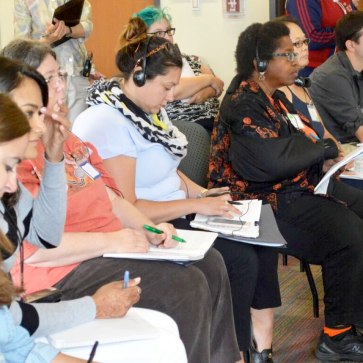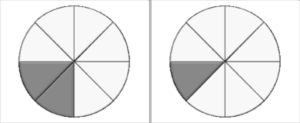# Conduct a Lesson Study Cycle on Fractions

Lesson Study works best with rich subject content. This course offers step-by-step support to conduct a Lesson Study cycle on fractions, by providing fractions resources and research to examine.

15-20 hours

##### MATERIALS:Part 1 | What Is Challenging About Fractions?

Task 2 : Find two fractions between 1/2 and 1.

#### Discussion Questions:

• How did you solve the problem, and how might students solve the problem?
• What understandings and misunderstandings about fractions might this problem reveal?
• Look at the student solutions to Task 2. What do the student responses suggest that each student understands and does not understand about fractions?
• Do you notice any differences in the responses of students who used the basal textbook and the students who participated in the “Measure Up” curriculum?
Below are six examples of student work on this task.  Three are from students who used a basal textbook and three are from students who learned fractions in a measurement context (the “Measure Up” curriculum): Context 1: Basal Text
 Student Response Rationale Student 1  1/4     1/5 because 4 is bigger than 1 because 5 is bigger than 1 Student 2  3/4     7/8 are between 1/2 and 1.  Both are missing one part. Student 3   2/3     4/5 2 is more than 1 3 is more than 2 4 is more than 1 5 is more than 2
Student work from Work Session presented by Barbara Dougherty and Barbara Fillingim, NCTM Annual Meeting Research Presession, April 21, 2009, Washington D.C., reproduced by permission of first author. Context 2: “Measure Up” Curriculum (Measurement Context)
 Student Response Rationale Student 1   2/3    3/4 If there is a lot of parts, there are smaller pieces. So you have to use a lot of them to get close to 1. So 2 out of 3 parts is close to 1 and so is 3 out of 4 parts Student 2   5/8     7/8 If you have 8 parts, then 4 parts are 1/2 so 5 parts and 7 parts are more then (sic) 1/2. But there (sic) not 1 because you need all the parts. Student 3   5/9     6/10 What I did was think of the number of parts and then what is haf (sic). Then I added one to it.
The  “Measure Up” curriculum emphasizes use of units of length, area, and volume to explore basic mathematical ideas such as equivalence.  For example, students might compare two lengths by using a third length.  Students using this curriculum become very attuned to asking, “What is the unit?” since different units (such as a hexagon and six triangles) might be used to create equivalence.

## What Makes Fractions So Difficult?

There’s something weird about fractions.  If the numerator, which is the top, is the same, the bigger the denominator the smaller the fraction.  - Jordan, Grade 5 student

Like Jordan, many students feel that “there’s something weird about fractions.”  The three tasks in this section shed some light on students’ challenges with fractions.

Task 1 : Estimate the answer to 12/13+ 7/8. You will not have time to solve the problem using paper and pencil.

A) 1

B) 2

C) 19

D) 21

#### Discussion Questions:

• How did you solve the problem, and how might students solve the problem?
• What insights into fractions make quick work of solving this problem?
• Discuss why students chose each of the responses shown. Why do so many students find this problem difficult?
 Answer Options Percent of Age 13 Students Who Chose Answer 1 7% 2 24% 19 28% 21 27% I don’t know 14%
Post, T. R. (1981, May). Fractions: Results and implications from National Assessment.  The Arithmetic Teacher.

Task 3: Jim has 3/4 of a yard of string which he wishes to divide into pieces, each 1/of a yard long. How many pieces will he have?

A) 3

B) 4

C) 6

D) 8

#### Discussion Question:

• How did you solve the problem and how might students solve the problem?
• Look at the student solutions from a US national assessment and explain what 70% of responding 4th grade students might have been thinking when they answered the above question incorrectly.
 Answer Options Percent of Age 13 Students Who Chose Answer 3 19% 4 30% 6* 27% 8 21% No response 3%

## Common Challenges with Fractions

From solving the three different fraction tasks and considering student solutions, summarize your team’s insights so far about the question:

• What is difficult for students about fractions?

The table “What’s Hard About Fraction Number Sense” summarizes six challenges in building fraction number sense, with examples of student understanding of each. Read each challenge, imagine what your students might find difficult, and click the challenge to see examples.

Discuss as a team:

• Anything in the table that is puzzling or particularly interesting to you
• Anything that helps you understand student responses to the tasks 1-3 that you examined

Record in Section 3 of the Teaching-Learning Plan any insights you would especially like to remember related to student thinking about fractions.

### What's Hard About Fraction Number Sense? (PDF)

 Challenge in Understanding Fractions Example of Student Difficulty or Understanding A Fraction is a NumberA fraction represents an amount, not just pieces (such as 2 of 3 pieces of a pizza) or a situation (such as 2 of 3 shirts are red). When asked to put the fraction 2/3  on a number line, a student said “you can’t put it on a number line, because it’s two pieces out of three pieces, it’s not a number.” Or “ 2/3 is not a number, it’s two numbers.” [*] Fractions Can Be Greater than OneMay be difficult for students who have a strong image of a fraction as a piece of something. “You can’t have 6/5 because there’s only 5/5 in a whole.” Fractions Can Be PartitionedA whole can be divided into smaller and smaller equal parts.The same fractional quantity can be represented by different fractions. Difficulty seeing how to divide a whole into equal parts.Difficulty seeing that 1/2 is equal to 2/4, 3/6,4/8, 5/10 and so on. What the Denominator MeansDifferent units (such as 1/3  and 1/5) are different sizes.The more units a whole is partitioned into the smaller each one is.1/n fits exactly n times into the whole. Students add 1/3 + 1/5 and get 2/8, without realizing they are adding two different things (thirds and fifths) sort of like adding apples and hammers.Students may think “1/5 is bigger than 1/4 because 5 is bigger than 4.”Difficulty seeing that 1/3 fits in the whole 3 times, 1/4 fits in the whole 4 times.  Has trouble seeing that 3/3, 4/4 etc. equal 1. Knowing What is the WholeConstructing the whole when given a fractional part.Keeping track of the whole. Difficulty making the whole when you give them a fractional part, e.g.: “This paper is2/3; show me the whole.”Sees that the magnitude of a fraction depends on the magnitude of the whole (e.g., half of a small cookie is not the same as half of a large cookie)Confusion about whether the two drawings below together represent 3/8 of a pie or3/16 of a pie.Fraction SizeUnderstands that fraction size is determined by the (multiplicative) relationship between numerator and denominator - not just by the numerator, not just by the denominator, and not by the difference between numerator and denominator.Sees non-unit fraction as an accumulation of unit fractions. [A unit fraction has a numerator of 1; a non-unit fraction has a numerator other than 1.] May think 4/9 is bigger than 3/4 because 4 is bigger than 3 (comparing numerators), or 4/9 is bigger than 3/4 because 9 is bigger than 4 (comparing denominators), or 3/5 is the same size as 5/7 because the difference between the top and the bottom in both fractions is 2.Sees that equivalent fractions have the same multiplicative relationship between numerator and denominator. In 2/4, 4/8, 3/6, etc. denominator is two times numerator.Sees 5/8 is made up of five 1/8‘s or 5 times 1/8, that 9/8 is made up of 9 eighths or 9 times 1/8, etc.

[*] Kerslake, D. (1986). Fractions: Children’s strategies and errors. A report of the strategies and errors in Concepts in Secondary Mathematics and Science Project. Windsor, England: NFER-Nelson. Behr, M.J. & Post, T.R. (1992). Teaching rational numbers and decimal concepts. In T.R. Post (Ed.), Teaching mathematics in grades K-8, research-based methods (pp. 201-248). Boston: Allyn and Bacon.